# Metric geometry : Corradicales circumferences Beams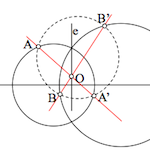By studying the equation of a circle in plane. saw a concrete determination is performed by determining three parameters in turn define the coordinates of its center and radius.

We can therefore say that in the plane there is a triply infinite set of circumferences, so if we set two restrictions, the parameters, we will be a purely infinite set which we call “beam circumferences

A beam circumferences is a simply infinite set of them.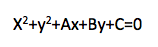Equation of the circle

We are interested in families of circles that share the same radical axis, and have their centers on a line (denominada base beam). This set will call le “beam of circles corradical”.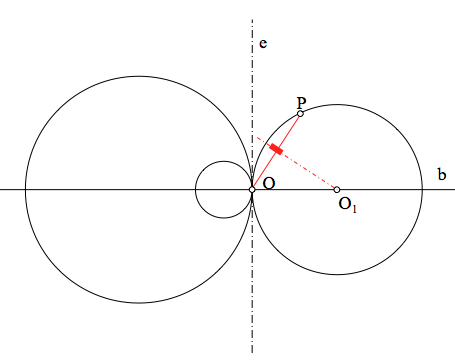Point O of intersection between the base line and the axis is called radical center beam.

A beam circumferences corradicales is a simply infinite set of circles with common radical axis (centers aligned in a straight base).

Reciprocally, given a circumference c and a straight and coplanar with it, can be found all circumferences (beam) having, with first, to the line and by radical axis.

There are three families can be distinguished in terms of the intersections of the members, or the radical position of the shaft relative to the circumferences. The classification can treat these circles evenly, adapting the basic constructs for each case:

### Haz eliptical

When the straight and, radical axis, is secant to the circle is called elliptical beam.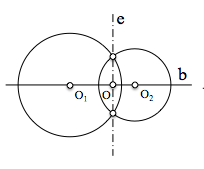The O beam center is internal to the circumference and therefore its power is negative. The radical axis also has zero power points (intersection of the circumferences) and positive power (Outside the circumferences)

All circles pass through two points on the radical axis called fundamental points beam.

The circumference of the smaller radius is on the diameter of the distance between main points.

### Make Parabolic

When the straight and, radical axis, is tangent to the circumference of the beam is called parabolic.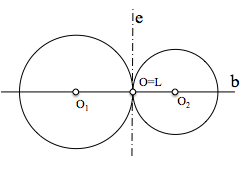The O beam center is the point of contact between all circumferences, and its power is zero (k=0). The other points of the radical axis have a positive value of the power with respect to the circumferences of the beam.

All beam circumferences are tangent to the radical axis in the center of the beam.

The circumference of the smaller radius is a point, Radio nulo, coincident with the center of the beam, so at this point is called limit point (O = L) .

### Haz Hiperbólico

When the straight and, radical axis, does not cut the beam circumference is called hyperbolic.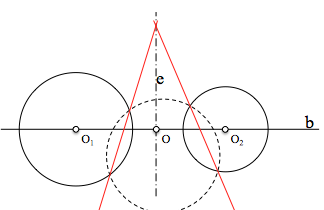The center of the beam is outside all the circles, so its power is not nula.Todos positive value of the radical axis points have values ​​greater than zero power.

Hyperbolic circumferences beam not intersecting

There circles of radio null which are known boundary points of the beam orby” (known as Poncelet points), we will see in detail to analyze this family of circles.

Metric Geometry

Note: This topic, developed during a summer by Professor D. Victorino García González, is dedicated to his memory.